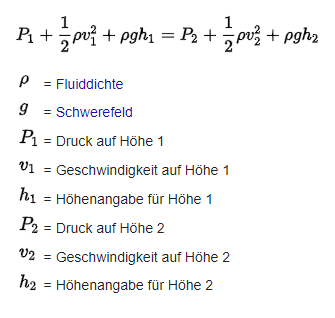Momentum Source as Force -> Velocity?

Hello There

I need to create a momentums source in order to get the right results.
Unfortenately i only have a force of 0.346 N and the geometry of the source moment given:
height = 0.1m, Length = 1.2m.

Is there any change to convert a force into a velocity so i can use it in SimScale?
I stuck with the Newton formula F = V* p * v / t (i dont know what the time is)
F = Force
V = Volume
p = Density
v = Velocity
t = time

any ideas?

regards
david

Hi
the force acting on a body can be normalized to a force coefficient.

Coeff = 2F/(p**Av²)
F = Froce
p = Density of the fluid
v = Velocity
A = reference Area

If you know the shape of your body you can then calculate the velocity for your force. There are many tables for coefficients for standard blunt bodies.

Another option could be to use the Bernoulli equation and using the pressure jumpYou could set P1 to 0 and v1 to Zero (or your free stream Velocity) and P2 to F*A
then calculate v2.

Do you have a link to your case so that we can have a look at it and see what approach fits your case best?

Best regards Sebastian

1 Like

Hi Sebastian
Thanks for the ideas.

When i use the equation with a cd of 0.001 (laminar flat plate) or 0.005 (turbulent flat plate) ive got high velocities of 70 m/s or 30 m/s. Both are unrealistic. Nevertheless the Bernoulli Equation makes much more sense (totally forget that one).

You could set P1 to 0 and v1 to Zero (or your free stream Velocity) and P2 to F*A
then calculate v2.

Setting P1 and/ or v1 to 0 leads to numerical problems. I solved it this way with P1 = Atmospheric pressure, v1 = 0.2 m/s (assumption, doesnt really matter), rgh is on both term 0 (no height difference). Since the fluid will get accelerated P2 must be smaller than P1. So the energie of the force goes into the velocity term increasing it, thus decreasing P2 by the amount of the force F/A. Ive got 2.2m/s for the velocity which could be possible.

I working on a validation case for calculating Draught Rating correctly. H Koskela1*and J Heikkinen https://www.irbnet.de/daten/iconda/CIB7276.pdf
I basically try to recreate the office room in the paper. So far i have problem getting the right velocity at the vicinity of the diffusor (it is just not good documented)
On the left picture (the green datapoints are from simscale)

Regards David

So far in the lower region of the room the results of SimScale look reasonable.
Where you able to find out why the difference in the top region is so off ?

Thank you and best regards Sebastian

Hi Sebastian
The difference in the upper region is due to the outlet boundary conditions. The boundary conditions arent good enough described in the papers. Unfortenately i dont think i will get it right, I already read 500 pages of source materials but it is confusing with different paper saying different things…
reards David

Hi David,

yes I know this problem. Unfortunately most of the papers do not well document the set up process and just discuss the results.

Anyway I wish you all the best for your project.

Best regards Sebastian